# Using Electricity

## Electrical Energy

The amount of electrical energy transferred to a device depends on:

1. The time it is used for
2. The power of the device (the rate at which it transfers energy)

Power is the rate of using energy. A 100W light bulb will use more electrical energy than a 60W bulb if they are both on for the same length of time

You need to remember the equation for power for your exam

### P = E ÷ t

P = power (W)

E = work done or energy transferred (J)

__t __= time (s)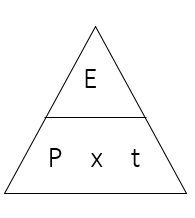## Worked Examples

Worked Example 1

Calculate the electrical energy used by the following devices:

An iPhone used for 3 hours (Power = 2W)

3 hours = 10800s

E = Pt = 2 x 10800 = 21,600J

A hairdryer used for 10 minutes (Power = 1500W)

10 mins = 600s

E = Pt = 1500 x 600 = 900,000J

A laptop used for 5 hours (Power = 50W)

5 hours = 18000s

E = Pt = 50 x 18000 = 900,000J

There are also two other equations for power that you need to memorise:

Power = Current x Voltage

P = I V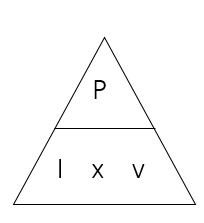Also, because Potential Difference = current x resistance…

Power = Current2__ x Resistance__

P = I2__ R__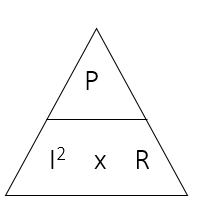Worked Example 2

What is the power of a 12V lamp with a current of 2A flowing through it?

Power = current x voltage

Power = 2 x 12

Power = 24W

Worked Example 3

A hairdryer has a resistance of 120Ω and draws a current of 4A. What is it’s power?

Power = current2 x resistance

Power = 42 x 120

Power = 1920W

## Fuses

A fuse protects an electrical device.

It contains a thin piece of wire which will melt and break the circuit if the current is too high.

This prevents damage to the device.

The rating of the fuse is the maximum current that the fuse can conduct.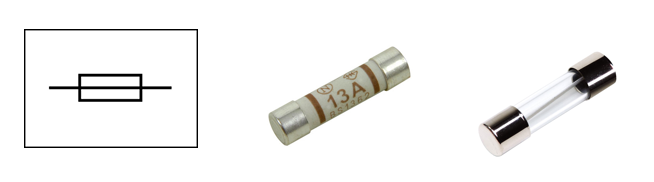Fuses are labelled with the maximum current they are designed to allow.

When choosing the fuse for a device you should always choose one with the ampage above the operating current of the device.

For example, if a kettle operates with an electrical current of 4.3A and the available fuses are 3A, 5A, and 13A, then you should choose the 5A fuse.

## UK Mains Electricity

1. AC supply
2. Frequency = 50 Hz (changes direction 50 times per second)
3. Potential Difference = 230V

## Generating Electricity

Electricity in generated in power stations using Electromagnetic Induction - When a magnet is moved in a coil of wire, current is induced in the coil.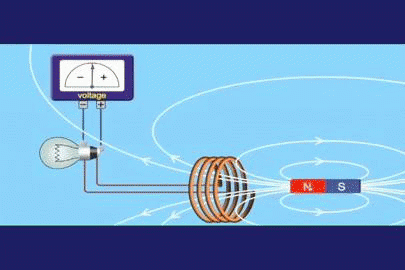Alternating Current (AC) is produced – this means the direction of the current changes direction repeatedly. In the UK, the mains electrical supply has a frequency of 50Hz – it changes direction 50 times per second.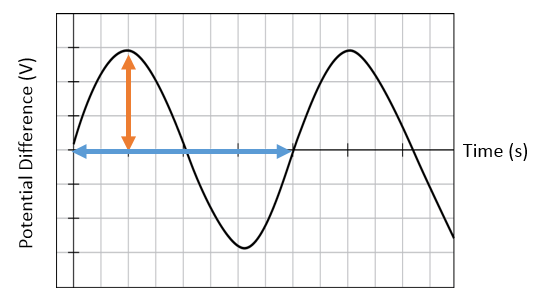1. Amplitude = peak voltage of the supply
2. Period = time for one complete wave
3. Frequency = number of waves per second
4. Frequency = 1 ÷ period

The size of the induced current can be increased by:

1. Increasing the strength of the magnet
2. Increasing the number of turns of wire on the coil
3. Placing an iron core inside the coil
4. Increasing the speed of the rotation

## Cables and Plugs

In the UK, most devices use a 3-core cable (the electrical cable has three separate wires inside)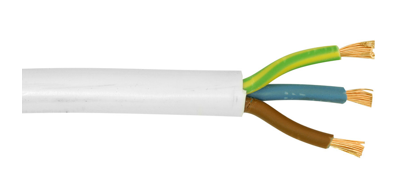Brown – live

Blue – neutral

Green/Yellow – earth

These are connected to 3 pin plugs:

The Earth pin is at the top with the live and neutral pins at the bottom. The fuse connected to live wire. There is a cable grip to keep the wires in place.

What does each wire do?

1. The live wire constantly alternates between a positive and negative voltage
2. The neutral wire is at 0V
3. The potential difference (or voltage) supplied to the device is the difference between the live and neutral wires
4. The earth wire is connected to any external metal parts of the device. If the live wire breaks and touches the case, the electric current would flow away through the earth wire, preventing the outside of the device becoming live

Double Insulation

1. Devices with no external metal parts do not need an earth wire
2. These devices are known as ‘double insulated’
3. The earth pin will often be made of plastic for double insulated devices

## National Grid

The National Grid is a network of wires and pylons connecting power stations and consumers together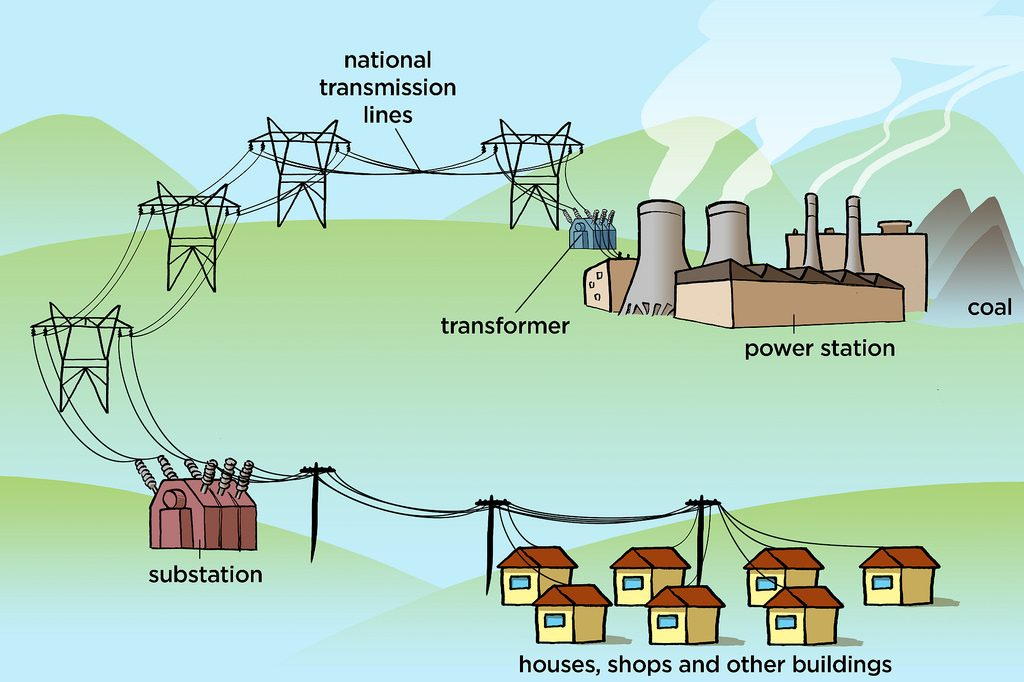Transformers

A transformer is a device which is used to increase or decrease voltage (potential difference)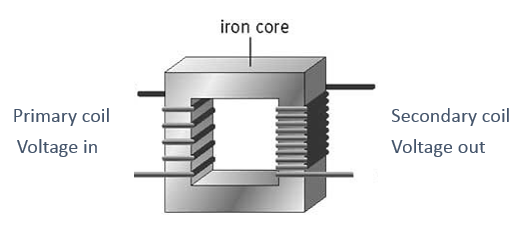1. A STEP UP transformer increases the voltage. It has more turns on the secondary coil.
2. A STEP DOWN transformer decreases the voltage. It has less turns on the secondary coil.

Every power station feeds into the grid and every consumer takes electricity from the grid. Transformers are used to step up voltage from power station to the grid and to step down voltage from the grid to homes (230V).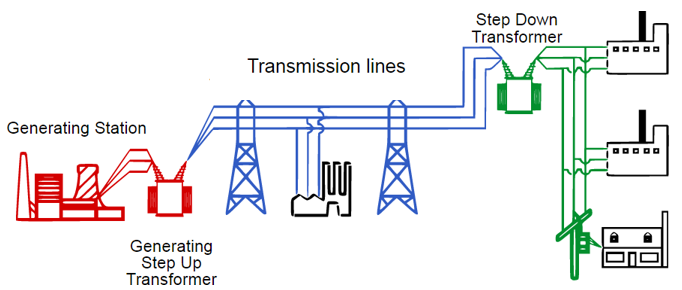High voltages are used between pylons (400,000 V) so that less energy is lost (high voltage = low current at constant power [P=IV])

What colour is the neutral wire?
blue
Which wire is attached to the fuse in a plug?
live
A 1.5W heater is used with the (230V) mains supply, how much current does it take?